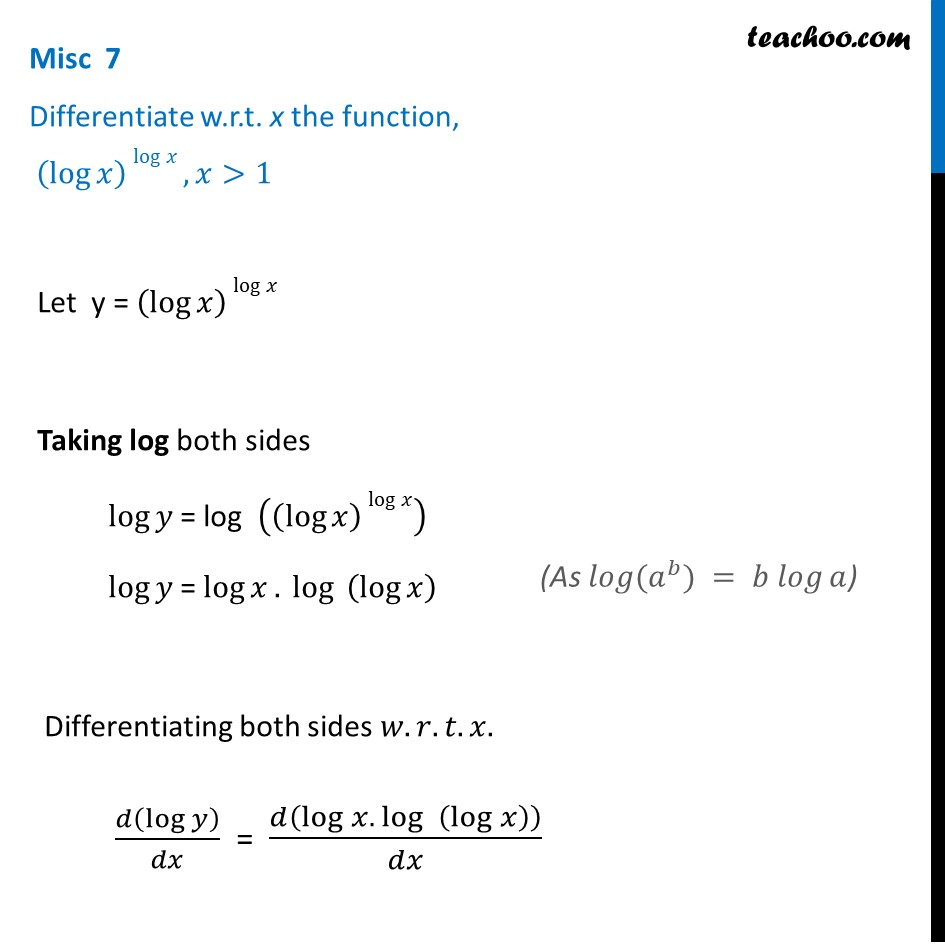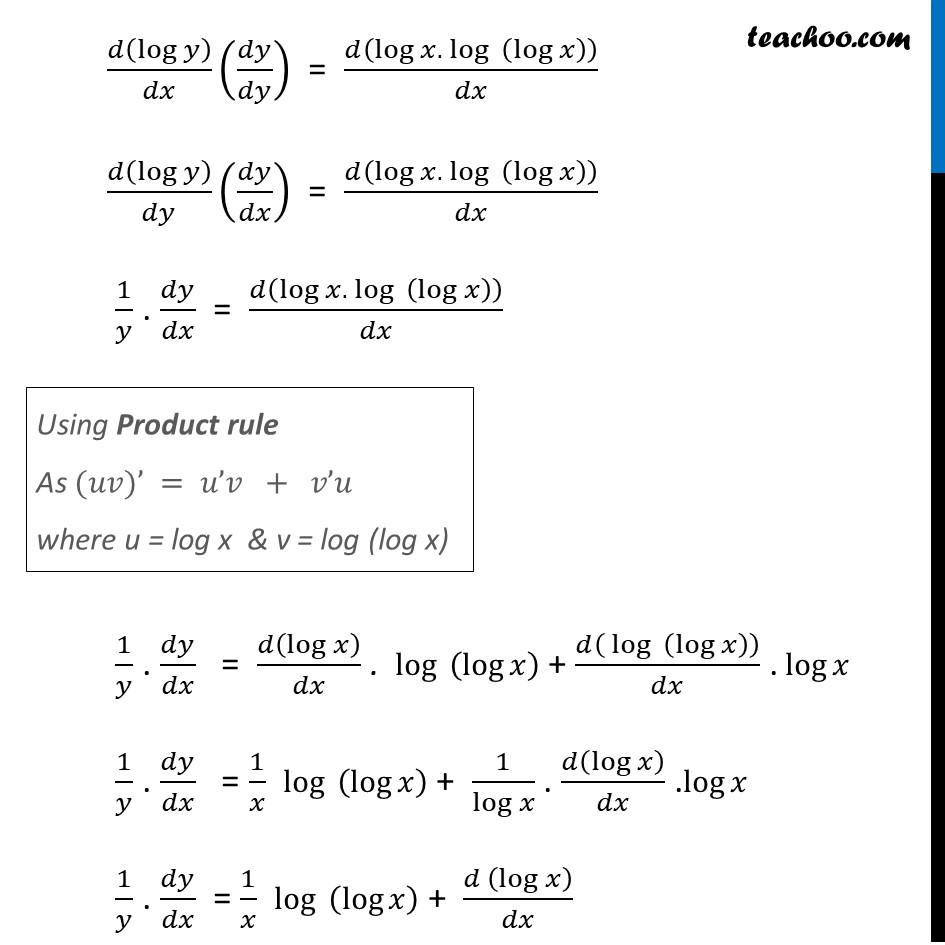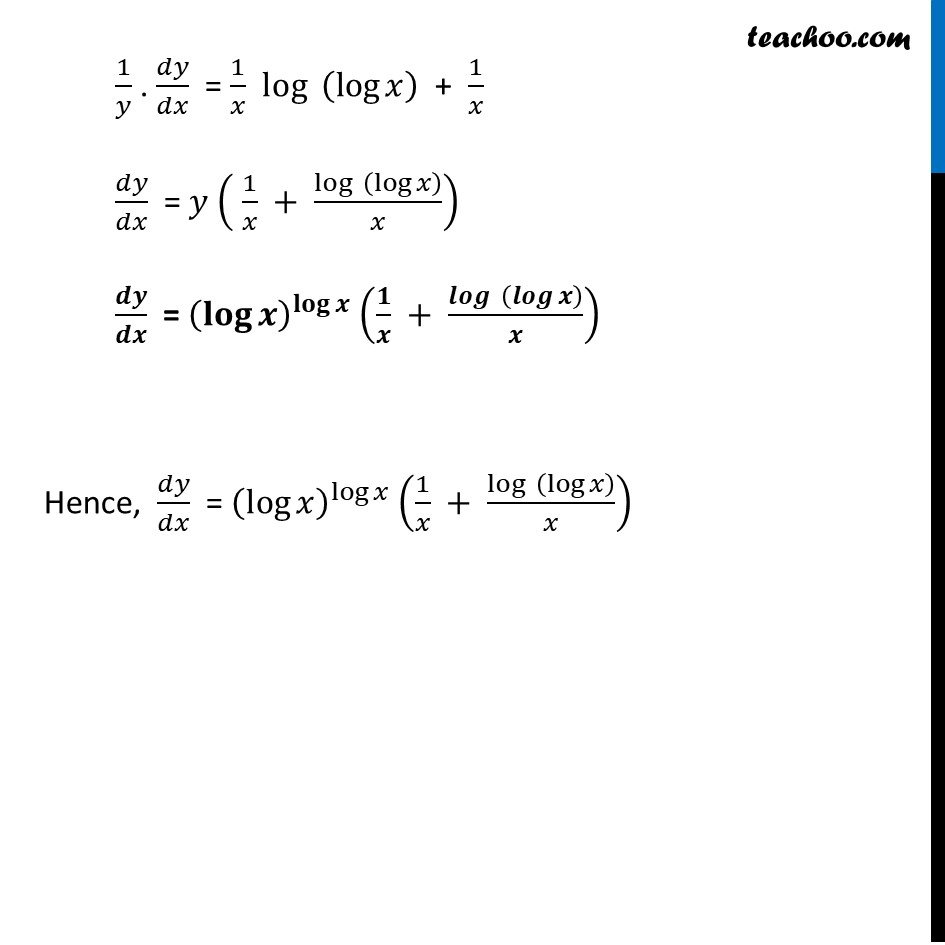Logarithmic Differentiation - Type 1

Chapter 5 Class 12 Continuity and Differentiability
Concept wiseLearn in your speed, with individual attention - Teachoo Maths 1-on-1 Class

### Transcript

Misc 7 Differentiate w.r.t. x the function, (log⁡𝑥 ) log⁡𝑥, 𝑥>1 Let y = (log⁡𝑥 ) log⁡𝑥 Taking log both sides log⁡𝑦 = log ((log⁡𝑥 ) log⁡𝑥 ) log⁡𝑦 = log⁡𝑥. 〖 log〗⁡〖 (log⁡𝑥 )〗 Differentiating both sides 𝑤.𝑟.𝑡.𝑥. 𝑑(log⁡𝑦 )/𝑑𝑥 = 𝑑(log⁡𝑥. 〖 log〗⁡〖 (log⁡𝑥 )〗 )/𝑑𝑥 (As 𝑙𝑜𝑔⁡(𝑎^𝑏) = 𝑏 𝑙𝑜𝑔⁡𝑎) 𝑑(log⁡𝑦 )/𝑑𝑥 (𝑑𝑦/𝑑𝑦) = 𝑑(log⁡𝑥. 〖 log〗⁡〖 (log⁡𝑥 )〗 )/𝑑𝑥 𝑑(log⁡𝑦 )/𝑑𝑦 (𝑑𝑦/𝑑𝑥) = 𝑑(log⁡𝑥. 〖 log〗⁡〖 (log⁡𝑥 )〗 )/𝑑𝑥 1/𝑦 . 𝑑𝑦/𝑑𝑥 = 𝑑(log⁡𝑥. 〖 log〗⁡〖 (log⁡𝑥 )〗 )/𝑑𝑥 1/𝑦 . 𝑑𝑦/𝑑𝑥 = 𝑑(log⁡𝑥 )/𝑑𝑥 . 〖 log〗⁡〖 (log⁡𝑥 )〗 + 𝑑(〖 log〗⁡〖 (log⁡𝑥 )〗 )/𝑑𝑥 .〖 log〗⁡𝑥 1/𝑦 . 𝑑𝑦/𝑑𝑥 = 1/𝑥 log⁡〖 (log⁡𝑥 )〗 + 1/log⁡𝑥 . 𝑑(log⁡𝑥 )/𝑑𝑥 . log⁡𝑥 1/𝑦 . 𝑑𝑦/𝑑𝑥 = 1/𝑥 log⁡〖 (log⁡𝑥 )〗 + (𝑑 (log⁡𝑥 ))/𝑑𝑥 Using Product rule As (𝑢𝑣)’ = 𝑢’𝑣 + 𝑣’𝑢 where u = log x & v = log (log x) 1/𝑦 . 𝑑𝑦/𝑑𝑥 = 1/𝑥 log⁡〖 (log⁡𝑥 )〗 + 1/𝑥 𝑑𝑦/𝑑𝑥 = 𝑦 ( 1/𝑥 + log⁡〖 (log⁡𝑥 )〗/𝑥) 𝒅𝒚/𝒅𝒙 = (𝐥𝐨𝐠⁡𝒙 )^𝐥𝐨𝐠⁡𝒙 (𝟏/𝒙 + 𝒍𝒐𝒈⁡〖 (𝒍𝒐𝒈⁡𝒙 )〗/𝒙) Hence, 𝑑𝑦/𝑑𝑥 = (log⁡𝑥 )^log⁡𝑥 (1/𝑥 + log⁡〖 (log⁡𝑥 )〗/𝑥)